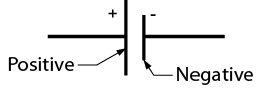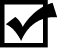# 9.12: Cells and Batteries

•• Camosun College
$$\newcommand{\vecs}{\overset { \rightharpoonup} {\mathbf{#1}} }$$ $$\newcommand{\vecd}{\overset{-\!-\!\rightharpoonup}{\vphantom{a}\smash {#1}}}$$$$\newcommand{\id}{\mathrm{id}}$$ $$\newcommand{\Span}{\mathrm{span}}$$ $$\newcommand{\kernel}{\mathrm{null}\,}$$ $$\newcommand{\range}{\mathrm{range}\,}$$ $$\newcommand{\RealPart}{\mathrm{Re}}$$ $$\newcommand{\ImaginaryPart}{\mathrm{Im}}$$ $$\newcommand{\Argument}{\mathrm{Arg}}$$ $$\newcommand{\norm}{\| #1 \|}$$ $$\newcommand{\inner}{\langle #1, #2 \rangle}$$ $$\newcommand{\Span}{\mathrm{span}}$$ $$\newcommand{\id}{\mathrm{id}}$$ $$\newcommand{\Span}{\mathrm{span}}$$ $$\newcommand{\kernel}{\mathrm{null}\,}$$ $$\newcommand{\range}{\mathrm{range}\,}$$ $$\newcommand{\RealPart}{\mathrm{Re}}$$ $$\newcommand{\ImaginaryPart}{\mathrm{Im}}$$ $$\newcommand{\Argument}{\mathrm{Arg}}$$ $$\newcommand{\norm}{\| #1 \|}$$ $$\newcommand{\inner}{\langle #1, #2 \rangle}$$ $$\newcommand{\Span}{\mathrm{span}}$$$$\newcommand{\AA}{\unicode[.8,0]{x212B}}$$

Cells and batteries convert chemical energy to electrical energy. They are used to provide power to portable electronic equipment. They may also be used for backup power in the event of a power failure.

The single cells shown in Figure 24 can produce 1 to 4 volts, depending on their compositions. For example, cells may be carbon-zinc, alkaline-manganese, nickel-cadmium, silver oxide, or lithium types.Figure $$\PageIndex{1}$$: Cells (CC BY-NC-SA; BC Industry Training Authority)

Note the polarity of the symbol for a cell shown in Figure Figure $$\PageIndex{2}$$.Figure $$\PageIndex{2}$$: Symbol for a cell (CC BY-NC-SA; BC Industry Training Authority)

Cells may be connected in series within a case to make up a battery. Both the 12-volt automotive battery and the 9-volt clip-in battery (Figure  $$\PageIndex{3}$$) have multiple cells inside the case. The symbol for a battery is composed of the number of symbols for cells corresponding to the number of cells in the battery (Figure $$\PageIndex{4}$$).Figure $$\PageIndex{3}$$: Batteries (CC BY-NC-SA; BC Industry Training Authority)Figure $$\PageIndex{4}$$: Symbols for batteries (CC BY-NC-SA; BC Industry Training Authority)Now complete the Learning Task Self-Test.